# Texas Go Math Grade 5 Lesson 1.4 Answer Key Compare and Order Decimals

Refer to our Texas Go Math Grade 5 Answer Key Pdf to score good marks in the exams. Test yourself by practicing the problems from Texas Go Math Grade 5 Lesson 1.4 Answer Key Compare and Order Decimals.

## Texas Go Math Grade 5 Lesson 1.4 Answer Key Compare and Order Decimals

Unlock the Problem

The table lists some of the mountains in the United States that are over two miles high. How does the height of Cloud Peak in Wyoming compare to the height of Boundary Peak in Nevada?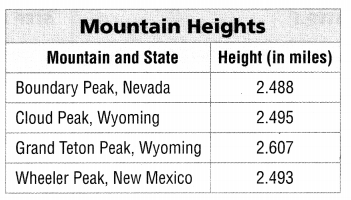One Way
Use place value.
Line up the decimal points. Start at the left. Compare the digits in each place-value position until the digits are different.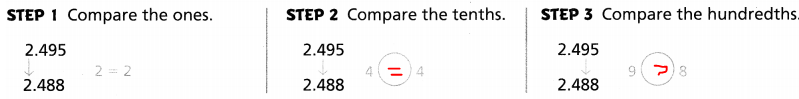Since 9 > 8, then 2.495 > 2.488, and 2.488 < 2.495.
So, the height of Cloud Peak is greater than the height of Boundary Peak.

Another Way
Use a place-value chart to compare
Compare the height of Cloud Peak to Wheeler Peak.Since 5 > 3, then 2.495 > 2.493, and 2.493 < 2.495.
So, the height of Cloud Peak is greater than the height of Wheeler Peak.

Math Talk
Mathematical Processes

Explain why it is important to line up the decimal points when comparing decimals.
Decimals extend the number system beyond the simple ‘hundreds, tens, units’ into ‘tenths of units’, ‘hundredths of units’ and so on. The most important rule to remember is to line up the decimal points in your calculation, ensuring that the decimal point in the answer also lines up with the decimal points above it

Example

Mount Whitney in California is 2.745 miles high, Mount Rainier in Washington * is 2.729 miles high, and Mount Harvard in Colorado is 2.731 miles high. Order the heights of these mountains from least to greatest. Which mountain has the least height? Which mountain has the greatest height?

STEP 1
Line up the decimal points. There are the same number of ones. Circle the tenths and compare.
2.745 Whitney
2.729 Rainier
2.731 Harvard
There are the same number of tenths.

STEP 2
Underline the hundredths and compare. Order from least to greatest.
2.745 Whitney
2.729 Rainier
2.731 Harvard
Since 2.729 < 2.731 < 2.745, the heights in order from least to greatest are 2.729, 2.731, 2.745.
So,
Rainier has the least height and Whitney has the greatest height.

Math Talk
Mathematical Processes

Explain why you do not have to compare the digits in the thousandths place to order the heights of the 3 mountains.
We know that,
The place-value of “Hundredths” is greater than the place-value of “Thousandths”
Hence, from the above,
We can conclude that
Since the place-value of hundredths is greater than the thousandths, you do not have to compare the digits in the thousandths place to order the heights of the 3 mountains

Share and Show

Question 1.
Use the place-value chart to compare the two numbers. What is the greatest place-value position where the digits differ?The given place-value chart is:Now,
From the given place-value chart,
We can observe that
The given decimal numbers are: 3.472 and 3.445
Now,
We know that,
The place-value of “Hundredths” is greater than the place-value of “Thousandths”
Now,
When we compare the place-values,
We can observe that
7 hundredths > 4 hundredths
Hence, from the above,
We can conclude that
The greatest place-value position where the digits differ is: Hundredths

Compare. Write <, >, or =.

Question 2.
4.563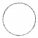4.536
The given decimal numbers are: 4.563 and 4.536
Now,
We know that,
The place-value of “Hundredths” is greater than the place-value of “Thousandths”
Hence,
4.563 > 4.536

Question 3.
5.6405.64
The given decimal numbers are: 5.640 and 5.64
Now,
From the given numbers,
We can observe that
All the place-values are the same
Hence,
5.640 = 5.64

Question 4.
8.6738.637
The given decimal numbers are: 8.673 and 8.637
Now,
We know that,
The place-value of “Hundredths” is greater than the place-value of “Thousandths”
Hence,
8.673 > 8.637

Name the greatest place-value position where the digits differ. Name the greater number.

Question 5.
3.579; 3.564
The given decimal numbers are: 3.579 and 3.564
Now,
We know that,
The place-value of “Hundredths” is greater than the place-value of “Thousandths”
Hence,
3.579 > 3.564

Question 6.
9.572; 9.637
The given decimal numbers are: 9.572 and 9.637
Now,
We know that,
The place-value of “Tenths” is greater than the place-value of “Hundredths”
Hence,
9.572 < 9.637

Question 7.
4.159; 4.152
The given decimal numbers are: 4.159 and 4.152
Now,
We know that,
The place-value of “Thousandths” is greater than the place-value of “Ten thousandths”
Hence,
4.159 > 4.152

Problem Solving

Order from greatest to least.

Question 8.
2.007; 2.714; 2.09; 2.97
The given decimal numbers are: 2.007, 2.714, 2.09, and 2.97
Now,
We know that,
The place-value of “Tenths” is greater than the place-value of “Hundredths” is greater than the “Thousandths”
Hence, from the above,
We can conclude that
The order of numbers from the greatest to the least is: 2.97, 2.714, 2.09, and 2.007

Question 9.
0.386; 0.3; 0.683; 0.836
The given decimal numbers are: 0.386, 0.3, 0.683, and 0.836
Now,
We know that,
The place-value of “Tenths” is greater than the place-value of “Hundredths” is greater than the “Thousandths”
Hence, from the above,
We can conclude that
The order of numbers from the greatest to the least is: 0.836, 0.683, 0.386, and 0.3

H.O.T. Algebra Find the unknown digit to make each statement true.

Question 10.
3.59 > 3.5 1 > 3.572
Let the unknown digit be x
Hence, from the above,
We can conclude that
The unknown digit that make the given statement true is:
3.59 > 3.581 > 3.572
Where,
x = 0, 1, 2, ……. 8

Question 11.
6.837 > 6.83 > 6.835
Let the unknown digit be x
Hence, from the above,
We can conclude that
The unknown digit that make the given statement true is:
6.837 > 6.836 > 6.835
Where,
x = 0, 1, 2, ……. 6

Question 12.
2.45 < 2. 6 < 2.461
Let the unknown digit be x
Hence, from the above,
We can conclude that
The unknown digit that make the given statement true is:
2.45 < 2.46 < 2.461
Where,
x = 0, 1, 2, ……. 4

Question 13.
Dawn keeps track of her softball batting average each year. The first year, her batting average is .783. The second year, her batting average is .81. In which year did she have the greater batting average?
It is given that
Dawn keeps track of her softball batting average each year. The first year, her batting average is .783. The second year, her batting average is .81
Now,
The given decimal numbers are: 0.783 and 0.81
Now,
We know that,
The place-value of “Tenths” is greater than the place-value of “Hundredths” is greater than the “Thousandths”
So,
0.81 > 0.783
Hence, from the above,
We can conclude that
In the second year, Dawn has the greatest batting average

Problem Solving

Use the table for 14-16.Question 14.
Use Math Language How does the height of Steele Mountain compare to the height of Blackburn Mountain? Compare the heights using words.
The given table is:Now,
From the given table,
We can observe that
The height of Steele Mountain is: 3.152 miles
The height of Blackburn Mountain is: 3.104 miles
Now,
We know that,
The place-value of “Tenths” is greater than the place-value of “Hundredths” is greater than the “Thousandths”
So,
3.152 > 3.104
Hence, from the above,
We can conclude that
The height of Steele Mountain is greater than the height of Blackburn Mountain

Question 15.
Write Math Explain how to order the height of the mountains from greatest to least.
The given table is:Now,
We know that,
The place-value of “Tenths” is greater than the place-value of “Hundredths” is greater than the “Thousandths”
So,
The order of the heights from the greatest to the least is:
3.152 > 3.134 > 3.104
Hence, from the above,
We can conclude that
The order of the height of mountains from the greatest to the least is:
3.152 miles > 3.134 miles > 3.104 miles

Question 16.
Multi-Step What if the height of Blackburn Mountain were 0.05 mile greater. Would it then be the mountain with the greatest height? Explain.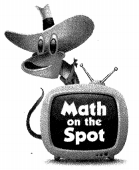The given table is:Now,
From the given table,
We can observe that
The height of the Blackburn Mountain is: 3.104 miles
The greatest height is: 3.152 miles
So,
The increased height of  Blackburn Mountain = 0.05 + 3.104
= 3.154 miles
So,
3.154 miles > 3.152 miles
Hence, from the above,
We can conclude that
With the height of Blackburn Mountain 0.05 miles greater, Blackburn Mountain will have the greatest height

Question 17.
The length of a piece of plastic for a science kit needs to be greater than 22.4 inches and less than 22.5 inches. Which length of plastic can be used?
A. 22.35 in
B. 22.51 in
C. 22.47 in.
D. 22.40 in.
It is given that
The length of a piece of plastic for a science kit needs to be greater than 22.4 inches and less than 22.5 inches.
So,
The numbers ca also be written as: 22.40 inches and 22.50 inches
Hence, from the above,
We can conclude that
The length of the plastic that can be used is: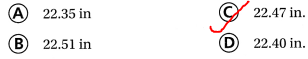Question 18.
Louis is comparing the numbers 8.402 and 8.451. What is the least place value he needs to compare to decide which number is greater?
A. ones
B. tenths
C. hundredths
D. thousandths
It is given that
Louis is comparing the numbers 8.402 and 8.451
Now,
The given numbers are: 8.402 and 8.451
Now,
We know that,
The place-value of “Tenths” is greater than the place-value of “Hundredths” is greater than the “Thousandths”
So,
We have to compare the hundredths place value to decide which number is greater
Hence, from the above,
We can conclude that
The least place value that he needs to compare to decide which number is greater is: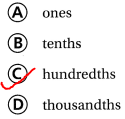Question 19.
Multi-Step Melinda compares four numbers. Which shows the numbers from least to greatest?
A. 3.04; 3.10; 3.529; 3.685
B. 3.10; 3.04; 3.529; 3.685
C. 3.10; 3.04; 3.685; 3.529
D. 3.04; 3.685; 3.529; 3.10
It is given that
Melinda compares four numbers
Now,
We know that,
The place-value of “Tenths” is greater than the place-value of “Hundredths” is greater than the “Thousandths”
Hence, from the above,
We can conclude that
The numbers that have the order from the least to the greatest is: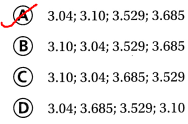Texas Test Prep

Question 20.
Mount Logan in the Yukon is 3.702 miles high. Mount McKinley in Alaska is 3.848 miles high and Pico de Orizaba in Mexico is 3.571 miles high. Order these mountains by height from greatest to least.
A. Logan, McKinley, Pico de Orizaba
B. McKinley, Logan, Pico de Orizaba
C. Pico de Orizaba, Logan, McKinley
D. Logan, Pico de Orizaba, McKinley
It is given that
Mount Logan in the Yukon is 3.702 miles high. Mount McKinley in Alaska is 3.848 miles high and Pico de Orizaba in Mexico is 3.571 miles high
Now,
The given decimal numbers are: 3.702, 3.848, and 3.571
Now,
We know that,
The place-value of “Tenths” is greater than the place-value of “Hundredths” is greater than the “Thousandths”
So,
The order of the numbers from the greatest to the least is:
3.848 > 3.702 > 3.571
Hence, from the above,
We can conclude that
The height of the mountains fro the least to the greatest is:### Texas Go Math Grade 5 Lesson 1.4 Homework and Practice Answer Key

Compare. Write <, >, or =.

Question 1.
9.239.32
The given decimal numbers are: 9.23 and 9.32
Now,
We know that,
The place-value of “Tenths” is greater than the place-value of “Hundredths”
Hence,
9.23 < 9.32

Question 2.
7.207.2
The given decimal numbers are: 7.20 and 7.2
Now,
We know that,
The number that has the last digit zero and the same number that does not have the last digit zero are the same
Hence,
7.20 = 7.2

Question 3.
1.9941.493
The given decimal numbers are: 1.994 and 1.493
Now,
We know that,
The place-value of “Tenths” is greater than the place-value of “Hundredths”
Hence,
1.994 > 1.493

Order from greatest to least.

Question 7.
7.081; 7.81; 7.002; 7.14
The given decimal numbers are: 7.081, 7.81, 7.002, and 7.14
Now,
We know that,
The place-value of “Tenths” is greater than the place-value of “Hundredths” is greater than the “Thousandths”
Hence, from the above,
We can conclude that
The order of numbers from the greatest to the least is: 7.81, 7.14, 7.081, and 7.002

Question 8.
1.001; 1.1; 1.403; 1.078
The given decimal numbers are: 1.001, 1.1, 1.403, and 1.078
Now,
We know that,
The place-value of “Tenths” is greater than the place-value of “Hundredths” is greater than the “Thousandths”
Hence, from the above,
We can conclude that
The order of numbers from the greatest to the least is: 1.403, 1.1, 1.078, and 1.001

Question 9.
0.04; 0.5; 0.021; 0.133
The given decimal numbers are: 0.04, 0.5, 0.021, 0.133
Now,
We know that,
The place-value of “Tenths” is greater than the place-value of “Hundredths” is greater than the “Thousandths”
Hence, from the above,
We can conclude that
The order of numbers from the greatest to the least is: 0.5, 0.133, 0.040, and 0.021

Question 10.
4; 4.022; 4.002; 4.221
The given decimal numbers are: 4, 4.022, 4.002, and 4.221
Now,
We know that,
The place-value of “Tenths” is greater than the place-value of “Hundredths” is greater than the “Thousandths”
Hence, from the above,
We can conclude that
The order of numbers from the greatest to the least is: 4.221, 4.022, 4.002, and 4

Problem Solving

Find the unknown digit to make each statement true,

Question 11.
5.345 > 5.34  > 5.343
Let the unknown digit be x
Hence, from the above,
We can conclude that
The unknown digit that make the given statement true is:
5.345 > 5.344 > 5.343
Where,
x = 0, 1, 2, 3, and 4

Question 12.
8.25 < 8. 6 < 8.361
Let the unknown digit be x
Hence, from the above,
We can conclude that
The unknown digit that make the given statement true is:
8.25 < 8.26 < 8.361
Where,
x = 0, 1, 2

Question 13.
6.48 > 6.4 1 > 6.470
Let the unknown digit be x
Hence, from the above,
We can conclude that
The unknown digit that make the given statement true is:
6.48 > 6.471 > 6.470
Where,
x = 0, 1, 2, ……. 7

Question 14.
Jack travels 2.45 miles to school. Wanda travels 2.31 miles to school. Compare the distances using words.
It is given that
Jack travels 2.45 miles to school. Wanda travels 2.31 miles to school
Now,
We know that,
The place-value of “Tenths” is greater than the place-value of “Hundredths” is greater than the “Thousandths”
So,
2.45 > 2.31
Hence, from the above,
We can conclude that
Jack travels more miles than Wanda to school

Question 15.
In a jumping contest, Marcus jumped 1.02 meters, Gustavo jumped 1.29 meters, and Loreena jumped 1.202 meters. Who won the jumping contest?
It is given that
In a jumping contest, Marcus jumped 1.02 meters, Gustavo jumped 1.29 meters, and Loreena jumped 1.202 meters
Now,
The given decimal numbers are: 1.02, 1.29, and 1.202
Now,
We know that,
The place-value of “Tenths” is greater than the place-value of “Hundredths” is greater than the “Thousandths”
So,
1.29 > 1.202 > 1.02
Hence, from the above,
We can conclude that
Gustavo won the jumping contest

Lesson Check

Question 16.
Which number has the greatest value?
A. 9.382
B. 9.47
C. 9.09
D. 9.7
We know that,
The place-value of “Tenths” is greater than the place-value of “Hundredths” is greater than the “Thousandths”
Hence, from the above,
We can conclude that
The number that has the greatest value is: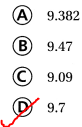Question 17.
Kerry is comparing the numbers 9.207 and 9.210. What is the least place value she, needs to compare to decide which number is greater?
A. ones
B. thousandths
C. hundredths
D. tenths
It is given that
Kerry is comparing the numbers 9.207 and 9.210
Now,
We know that,
The place-value of “Tenths” is greater than the place-value of “Hundredths” is greater than the “Thousandths”
So,
We have to compare the “Hundredths” place value to find the greatest number in the given decimal numbers
Hence, from the above,
We can conclude that
The least place value Kerry needs to compare to decide which number is greater is:Question 18.
Which number has the least value?
A. 7.034
B. 7.304
C. 7.403
D. 7.003
We know that,
The place-value of “Tenths” is greater than the place-value of “Hundredths” is greater than the “Thousandths”
Hence, from the above,
We can conclude that
The number that has the least value is: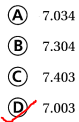Question 19.
In 5.820, what is the place-value position of the 0?
A. thousandths
B. tenths
C. hundredths
D. ones
The given decimal number is: 5.820
Now,
The representation of 5.820 in the place value chart is:Hence, from the above,
We can conclude that
The place value position of 0 in 5.820 is:Question 20.
Multi-Step Jenna compares the lengths of her pet fish. The table shows the lengths of the fish. Which shows the lengths in order from greatest to least?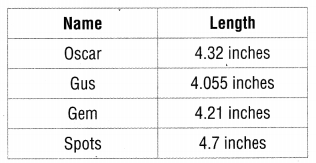A. Spots, Oscar, Gus, Gem
B. Oscar, Spots, Gem, Gus
C. Gus, Oscar, Gem, Spots
D. Spots, Oscar, Gem, Gus
It is given that
Jenna compares the lengths of her pet fish. The table shows the lengths of the fish
Now,
The given table is:Now,
We know that,
The place-value of “Tenths” is greater than the place-value of “Hundredths” is greater than the “Thousandths”
So,
From the given table,
We can observe that
The order of the numbers that are from the greatest to the least is:
4.7 > 4.32 > 4.21 > 4.055
So,
The order of the lengths of Jenna’s pet fish is:
Sports > Oscar > Gem > Gus
Hence, from the above,
We can conclude that
The lengths of Jenna’s pet fishes in order from the least to the greatest is: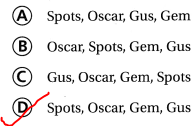Question 21.
Multi-Step Look at the table in Question 20. What if Gem grew 0.5 inches. Which would then show the lengths in order from greatest to least?
A. Gem, Spots, Gus, Oscar
B. Gus, Oscar, Gem, Spots
C. Gem, Spots, Oscar, Gus
D. Spots, Oscar, Gem, Gus
The given table is:Now,
The present length of Gem = (The old length of gem) + 0.5
= 0.5 + 4.21
= 4.71 inches
So,
The order of the lengths of Jenna’s pet fish according to the new size of Gem is:
Gem > Sports > Oscar > Gus
Hence, from the above,
We can conclude that
The order of the lengths of pet fish from the greatest to the least after the increase of Gem’s length by 0.5 inches is: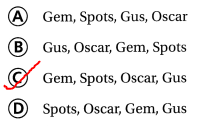Scroll to Top